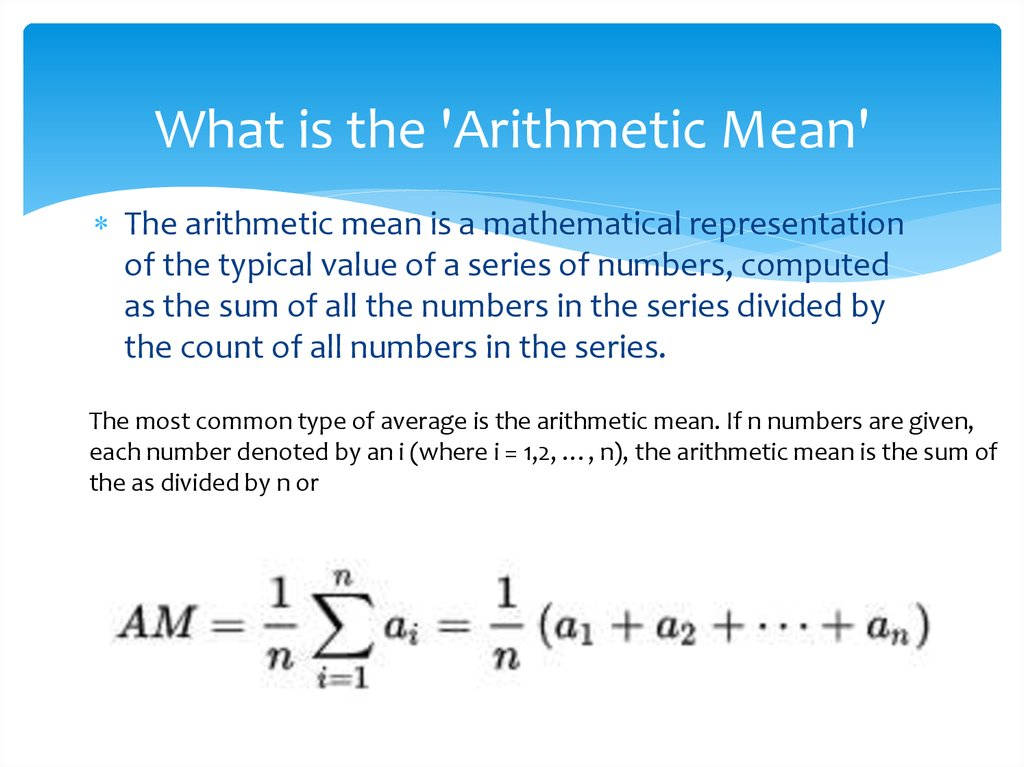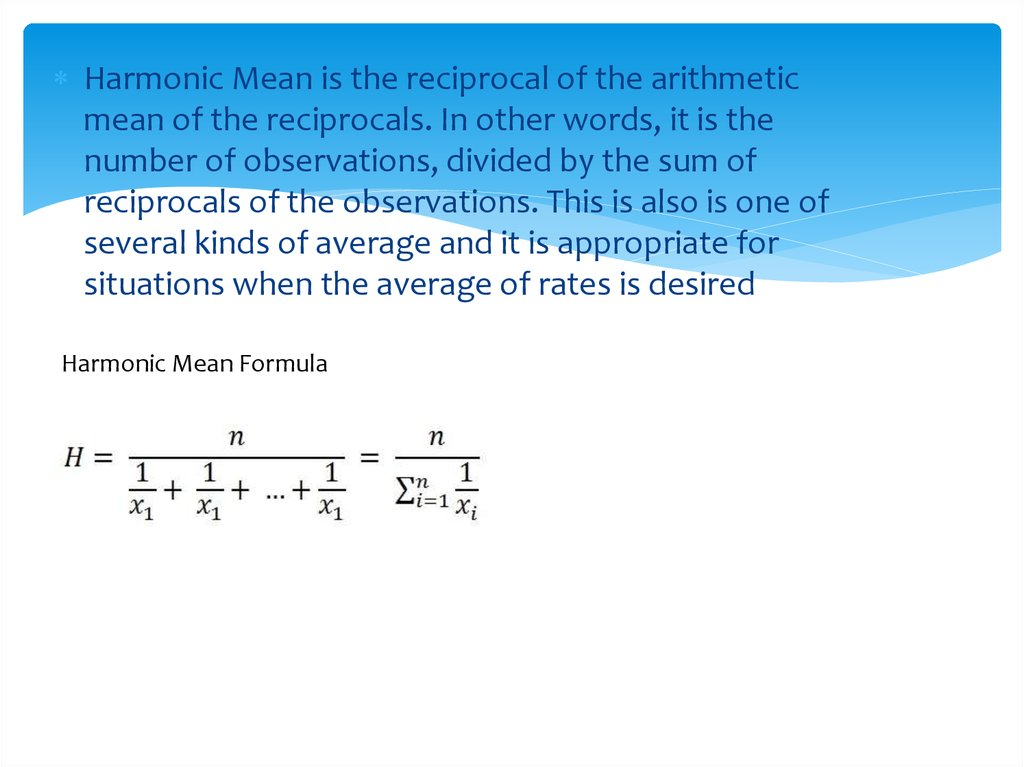# The mean

## 2. What is the 'Arithmetic Mean'

The arithmetic mean is a mathematical representation
of the typical value of a series of numbers, computed
as the sum of all the numbers in the series divided by
the count of all numbers in the series.
The most common type of average is the arithmetic mean. If n numbers are given,
each number denoted by an i (where i = 1,2, …, n), the arithmetic mean is the sum of
the as divided by n or

## 3.

Harmonic Mean is the reciprocal of the arithmetic
mean of the reciprocals. In other words, it is the
number of observations, divided by the sum of
reciprocals of the observations. This is also is one of
several kinds of average and it is appropriate for
situations when the average of rates is desired
Harmonic Mean Formula

## 4. What is the 'Geometric Mean'

The geometric mean is the average of a set of
products, the calculation of which is commonly used
to determine the performance results of an
investment or portfolio.
The geometric mean is always less than or equal to the
arithmetic mean

## 5. RMS

In statistics and its applications, the root mean square
(abbreviated RMS or rms) is defined as the square root of
mean square (the arithmetic mean of the squares of a set
of numbers). The quadratic mean, is a statistical measure
of the magnitude of a varying quantity. The RMS is also
known as the quadratic mean and is a particular case of
the generalized mean with exponent 2. RMS can also be
defined for a continuously varying function in terms of an
integral of the squares of the instantaneous values during
a cycle.

## 6.

Root mean square or quadratic mean of
collection of real numbers X1…..,Xn is defined to
be